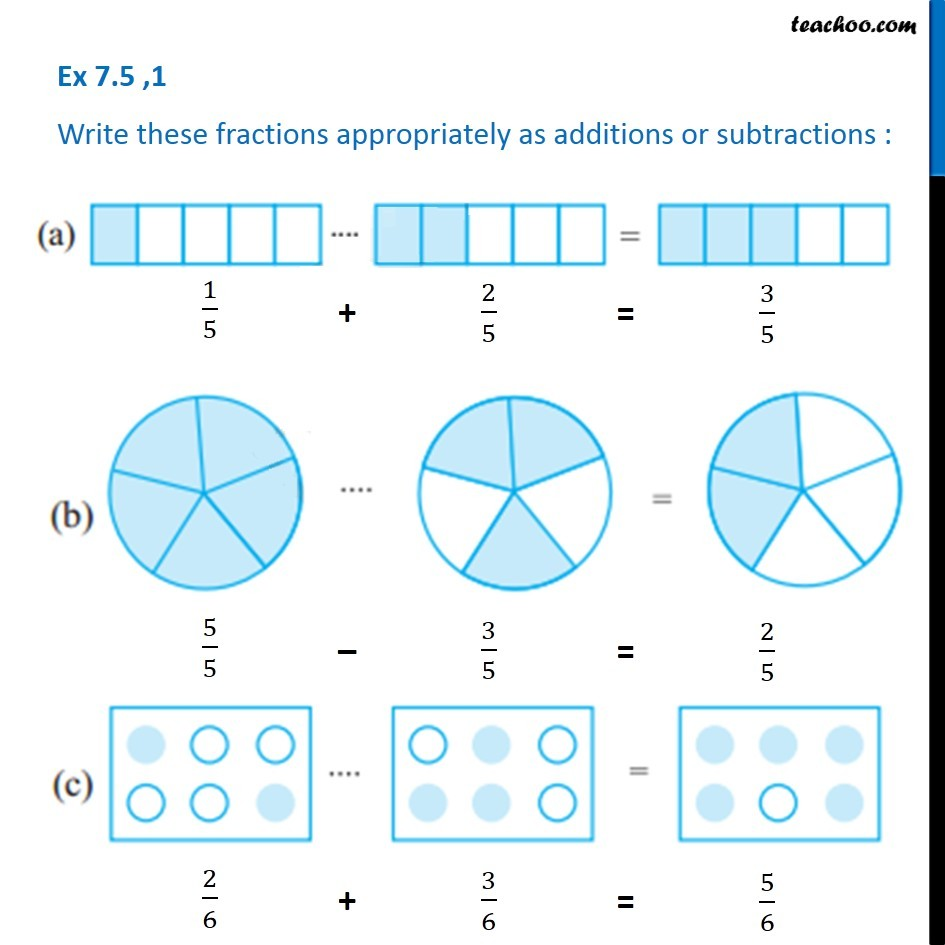1. Chapter 7 Class 6 Fractions
2. Serial order wise
3. Ex 7.5

Transcript

Ex 7.5 ,1 - Chapter 7 Class 6 Fractions - NCERT Books Write these fractions appropriately as additions or subtractions: From figure, we first write fractions, and then write addition or subtraction symbol (a) 1/5 + 3/5 = 2/5 (b) 5/5 - 3/5 = 2/5 (c) 2/6 + 3/6 = 5/6

Ex 7.5

Chapter 7 Class 6 Fractions
Serial order wise